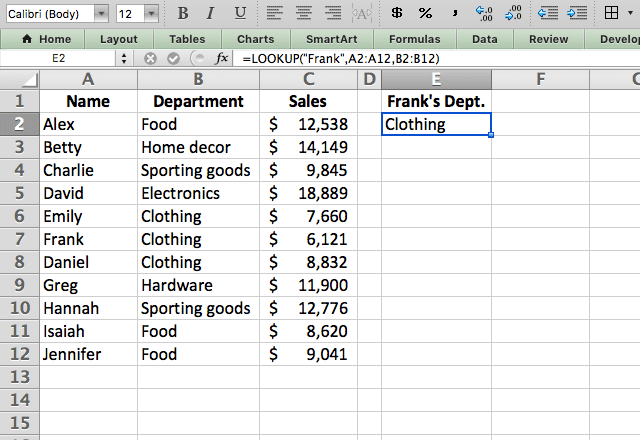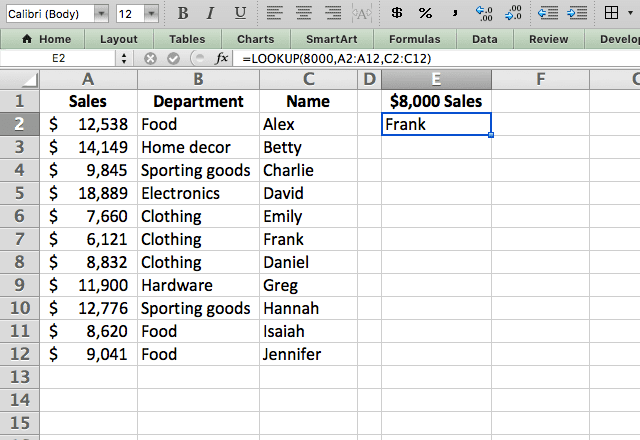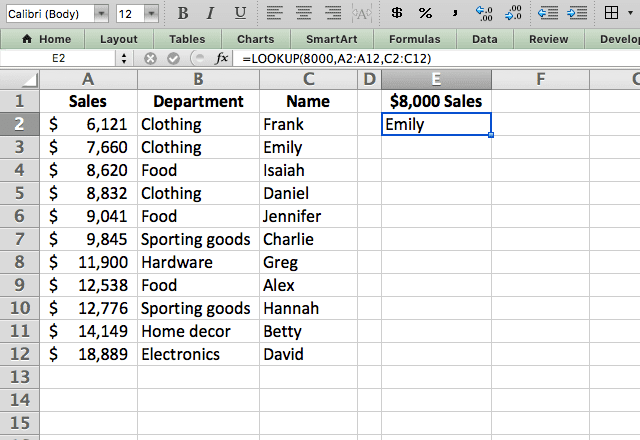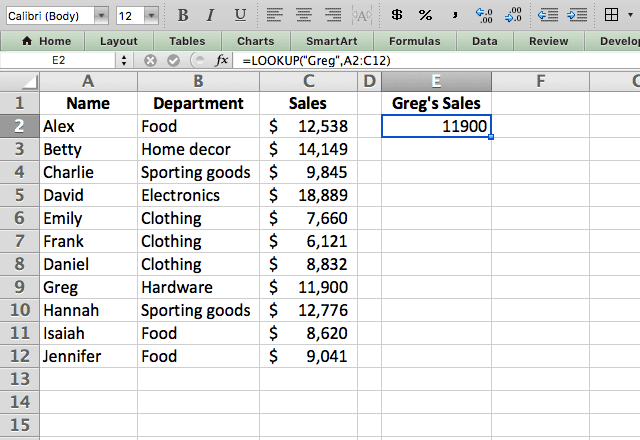# How to use the LOOKUP function in ExcelA common task in Excel is searching your spreadsheet for certain values, and using a function to do this and return a corresponding value can be very useful. The LOOKUP function in Excel is one way to do this. While the VLOOKUP and HLOOKUP functions are more powerful, the LOOKUP function is great for quick and simple lookups to keep your spreadsheet running smoothly.

### Syntax

The LOOKUP function has two forms: vector form and array form.

#### Vector form

This is the form of the function that you’ll typically use. You specify the lookup value and the search and results vectors, and the function will find the best match.>/p>

=LOOKUP(lookup_value, lookup_vector, [result_vector])
• lookup_value is the item you are searching for
• lookup_vector is the range you want to search in. This can only be one column or row
• result_vector is an optional input. It’s the range you want to get the result from

#### Array form

This form is less common but can be just as useful.

=LOOKUP(lookup_value, array)
• lookup_value is the item you are searching for
• array is the range you want to search and find the result from. It will search the first column/row for the lookup_value and pull the answer from the last column/row

### How to use the LOOKUP function in Excel

Now that you’ve seen the syntax, I’ll show some examples of the Excel LOOKUP function in action.

I’ll start with the vector form since this is the most common. Here I have some data from a department store, and I’m using the function to find Frank’s department.I enter “Frank” as the search key, and tell the function to search in A2:A12. I also instruct the function to return the corresponding answer from B2:B12.

I do this with the formula =LOOKUP(“Frank”,A2:A12,B2:B12).

Note that the two vectors are the same length – if they aren’t, you’ll get an error!

#### Unsorted data

When you use the LOOKUP Excel function, your data needs to be sorted in ascending order. The LOOKUP function provides an approximate match to your search value when there’s no exact match.

To do this, it needs sorted data. In this example, I demonstrate what happens when the data is not sorted.Here, I’m searching for the person with sales closest to \$8,000. By typing =LOOKUP(8000,A2:A12,C2:C12) I can make the LOOKUP function do just that.

But you can see here that the answer is wrong. The reason for that is my data isn’t sorted, so the function can’t tell what the right answer is!

I can fix that problem by sorting the data by sales in ascending order. Now I get the correct answer!

Note that the LOOKUP function will always return the value lower than the search value when there’s no exact match. Even if Isaiah had \$8,001 in sales, it would still return Emily because she’s the closest to \$8,000 without surpassing it!#### Array format

You’ve now seen how the LOOKUP function in Excel works in vector format. As I mentioned previously, that’s the more common format.

But you also have the option of using array format. The difference here is you only need to specify a lookup_value and a single array.

In the example below, I find Greg’s sales using the formula =LOOKUP(“Greg”,A2:C12).The benefit of the array format is you only have to specify one array, instead of two separate vectors.

The function will automatically search the first column (or row if it’s a horizontal array) for the lookup_value and return the result from the last column (or row).

### Tips for using the Excel LOOKUP function

• The LOOKUP function automatically searches for approximate matches. Unlike the VLOOKUP or HLOOKUP functions, you can’t specify an exact match
• The data must be sorted in ascending order, otherwise you’ll get incorrect results
• It will always return a value smaller or equal to the lookup_value, never larger. This is true even if a larger value is closer to the lookup_value than the smaller value
• The VLOOKUP (vertical lookup) and HLOOKUP (horizontal lookup) functions are more versatile and useful versions of LOOKUP
• LOOKUP is not case-sensitive